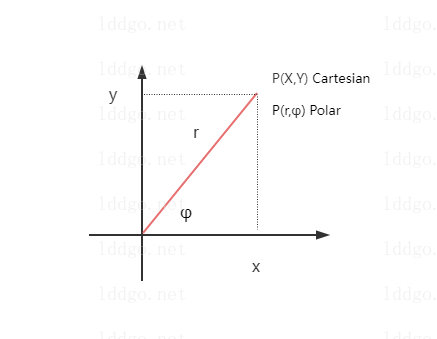•• ARTICLE
• STRING
• CONVERTER
• ENCRYPT
• NETWORK
• MORE
CHART
MATH
COORDINATE
IMAGE
• ARTICLE
STRING
CONVERTER
ENCRYPT
NETWORK
MORE
CHART
MATH
COORDINATE
IMAGEOnline Tools

# Cartesian and Polar Coordinate Converter Online

Related Tools
Cartesian and Polar Coordinate Converter Online-summary

The online cartesian coordinate and polar coordinate conversion tool supports the mutual conversion of Cartesian coordinate system coordinates and Polar coordinate system coordinates. The conversion supports batch operation, and the conversion results can be downloaded locally.

Cartesian and Polar Coordinate Converter Online-instructions

Online polar coordinate and Cartesian plane rectangular coordinate conversion tool, supporting polar coordinates (r, φ) Convert to and from Cartesian coordinates (X, Y).

1. Definition of Cartesian coordinate system : The origin is (0,0), the positive direction of the X-axis is left to right, and the positive direction of the Y-axis is 90° counterclockwise rotation of the X-axis. The positive direction of the X-axis points to the right and the positive direction of the Y-axis points top.
2. Definition of polar coordinate system : The origin is (0,0), and the polar axis is in the positive direction of the X-axis.
3. Coordinate system diagram :4. Formula for converting polar and Cartesian coordinates
polar coordinates → Cartesian coordinates
Cartesian coordinates → polar coordinates
Formula Description : The range of φ values is (-π, π], and the corresponding degree is (-180, 180]. atan2 is the arctangent function that has taken quadrants into account.
5. Input Coordinates : Enter the plane Cartesian coordinates (X, Y) or polar coordinates (r, φ). Use Comma-separated values, and enter one coordinate for each line. Supports input of multiple lines. XY coordinate format is X , Y. rφ coordinate format is r , φ.
6. Unit : Select the angle unit in the input or result, supporting degrees (°) and radians (rad).
7. Range : Select the range of values for the polar angle in the input or result polar coordinates. Supports (-180,180] or (-π, π], [0,360) or [0,2π).
8. Precision : The number of digits reserved after the decimal point of the conversion result. Optional. The range is [0,15].
9. XY → rφ : Convert Cartesian coordinates to polar coordinates (r is the radius coordinate, φ Is the angular coordinate).
10. rφ → XY : Convert polar coordinates to Cartesian coordinates.
11. Open File : Support opening UTF-8 encoded text files.
12. Input Coordinates Example :
a. Input Cartesian coordinates
1 , 1 -1 , 1 -1 , -1 1 , -1  b. Input polar coordinates( the unis is degree, angel range is (-180,180] )
1.414 , 45.000 1.414 , 135.000 1.414 , -135.000 1.414 , -45.000  c. Input polar coordinates( the unis is radian, angle range is [0, 2π) )
1.414 , 0.785 1.414 , 2.356 1.414 , 3.927 1.414 , 5.498